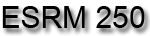Introduction to Geographic Information Systems in Forest Resources

Raster Analysis II

Discussion:

There are many different types of raster analysis available in ArcGIS. Here are just a few of the common analytical functions. More analytical functions on raster surfaces models will be dealt with in 3-D and Surface Modeling.

Importing data from generic raster files

Many datasets are available on the World Wide Web. Most of the raster datasets are in a generic format. Those formats that can be imported into ArcGIS are

• The ASCII raster file format
• The binary raster file format
• The USGS Digital Elevation Model (DEM) raster file format
• The US DMA DTED raster file format

The most common format you are likely to see is the USGS DEM. There is a page of Washington 10 and 30 m DEMs for USGS 7.5' quad sheet boundaries on a server in Geological Sciences.

Using ArcToolbox it is possible to import these data sets. The Spatial Analyst Extension must be activated in order to import raster data. Imported raster data will be converted to the ArcInfo raster grid data format.

Here, a USGS DEM was downloaded from the site in Geological Sciences and unzipped. Importing the grid is very straightforward using ArcGIS 's GUI.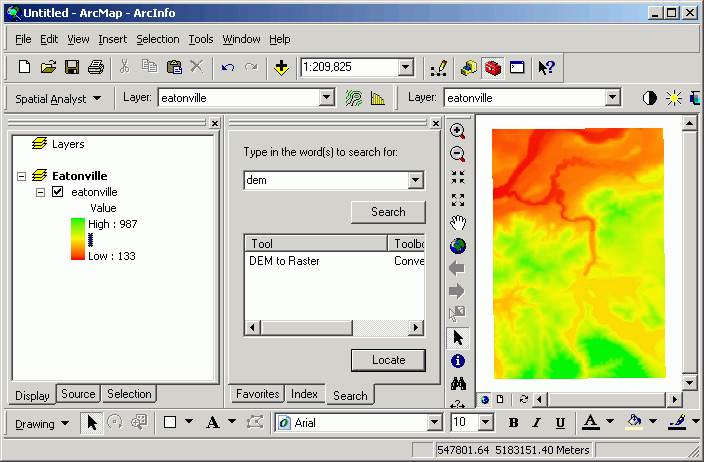The same basic process is used to import from the other raster interchange file formats.

Merging or mosaicking adjacent grids is used when your study area falls across several grids, and you wish to treat those grids as a single grid. This is commonly used when the data source is the USGS series of DEMs. Because DEMs are created and distributed as tiles, if your study area falls across several tiles, it is often necessary to merge these tiles together.

In this example, I have downloaded and imported the Elbe DEM as well as the Eatonville DEM. The following images show the grid created from mosaicking the two inputs (before [above], and after [below]).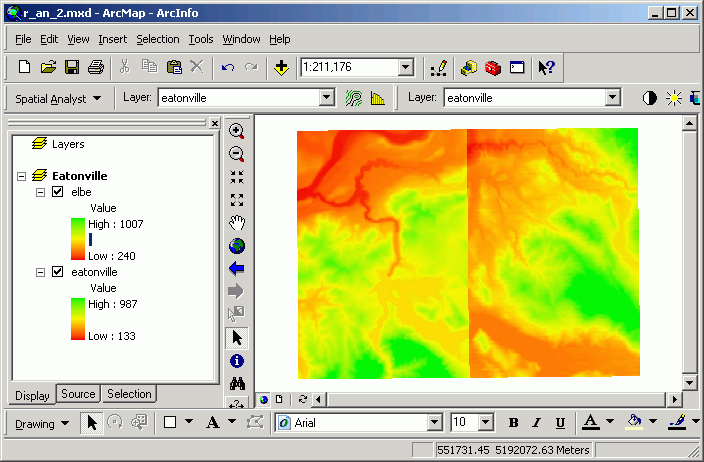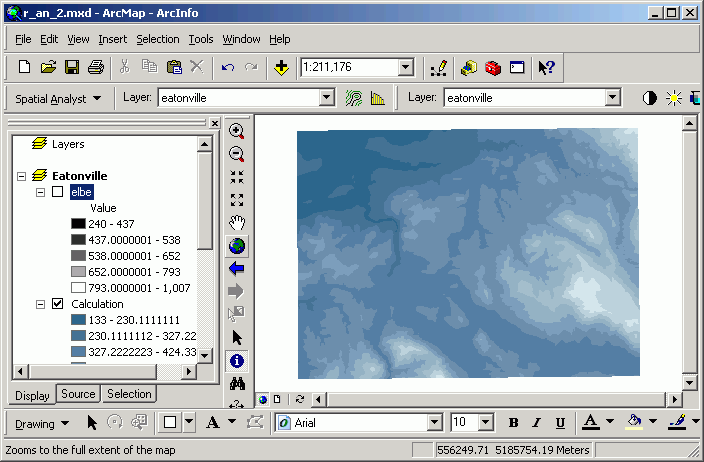Calculating distance surfaces and buffers

Distance surfaces are grids whose output value is the distance to the closest feature in the input layer. The input layer can be a selected set of any type of feature (point, line, polygon, or grid cell). Distance surfaces are calculated by using the Spatial Analyst> Distance menu choice in the Spatial Analyst toolbar.

Distance surfaces are similar to buffers in the vector world. The difference between vector buffering and creating distance grids is that the distance surface represents a continuous change in distance from the source as you move across the landscape, whereas the buffer analysis changes in user-defined quantized steps.

Here are the streams of Pack Forest and a distance surface created from them: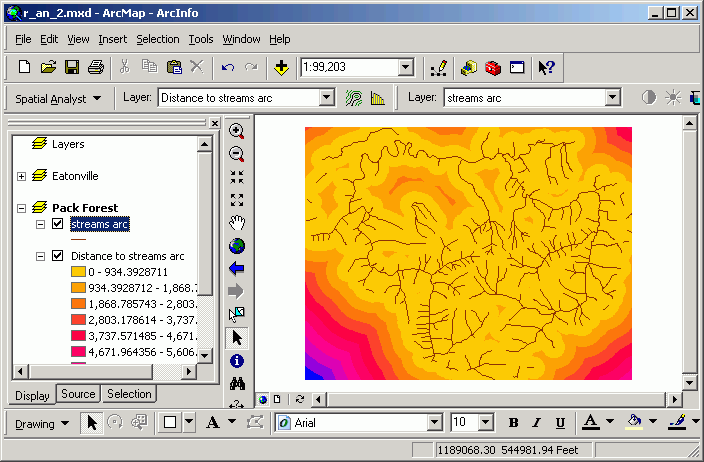A distance grid is calculated. Every cell in the output dataset is assigned cell value equal to the straight-line distance to the closest stream line feature. Those cells closest to the stream are light yellow in color, and those farthest away are blue (note the southwest corner). Note that this is different from a buffer, which only gives an "inside or outside" encoding of the output data set. Although the image shows "rings" of distance classes, the underlying data are continuous in value.

Determining proximity

Determining proximity is similar to calculating a distance surface, but rather than creating a continuous surface whose value is the distance to a feature, the proximity grid contains values in the cells for a corresponding value in the input feature attribute table. Each cell is coded with the closest feature's value from the input layer, rather than for the distance to features.

Proximity analysis uses as input the selected set of the active layer, and is available from the menu at Spatial Analyst > Distance > Allocation.

Here, proximity is calculated for some bird nest points. In the output, the value for any given cell in the output Allocation grid layer is the sequential ID number for the closest nest.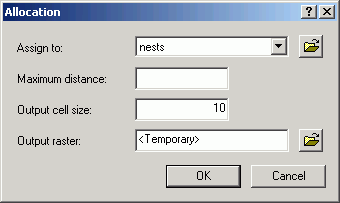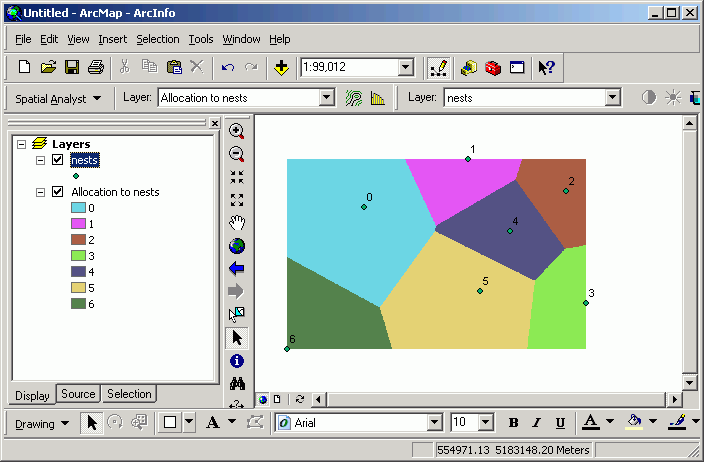Every cell is encoded for the value of the closest point. This means that, for example, anywhere within grid zone 5 is closer to point 5 than to any other point. The cells on the edge between zone 4 and 5 are equidistant to either point.

This technique is also known as Thiessen or Voronoi analysis.

Creating surfaces from point samples

Frequently point samples are taken to because it is too costly (either in terms of time or money) to sample an entire population. It is possible to generate interpolated surfaces based on point samples. The cells between the sampling points are given a value that represents a smooth transition of value between the sampling points. If you need an estimate of a value somewhere that you do not have a sampling point, you can get a grid value at that spot. Be careful here, because the assumption that values change smoothly across the landscape is not necessarily true! This type of analysis is well-suited to data that definitely do change gradually over a large area, such as precipitation. In any case, if your sampling points are spread too far apart, you may create an interpolated grid that does not capture local variations.

Here is a surface generated from the Pack Forest CFI plot centers using a Regularized Spline method. Red indicates low standing wood volume and green indicates high standing wood volume for conifer trees in 1994. Plot centers are also displayed here for illustration.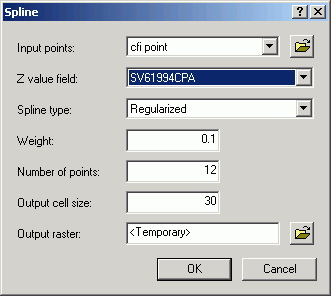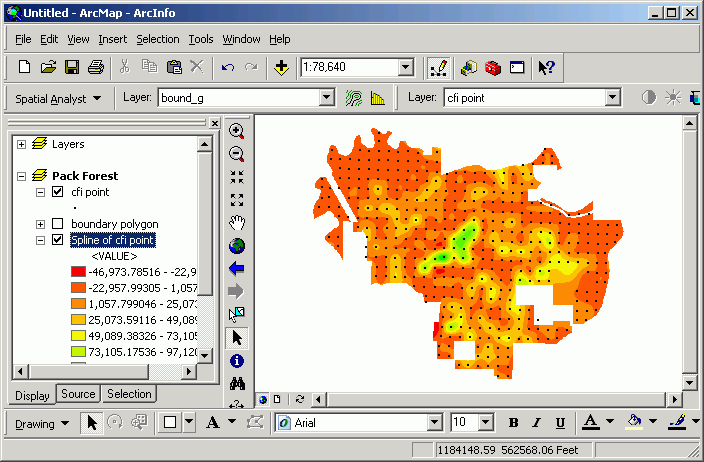There are a number of different options for creating surfaces from point samples. If you need to perform surface interpolation from points, you should read the help documents thoroughly.

Mapping contours

If you have data representing a continuous surface, it is possible to create single contour lines for a given grid cell value, or to create a whole group of contour lines at a regular interval. This can be of value if you wish to create a contour map of any continuously changing surface. Although digital vector elevation contours are available for some USGS quad sheets, many areas of the state have not been digitized yet. However, we do have complete statewide coverage for DEMs. These DEMs can be used to create contour lines that can be added to maps.

Here are contours made from 30 m DEMs: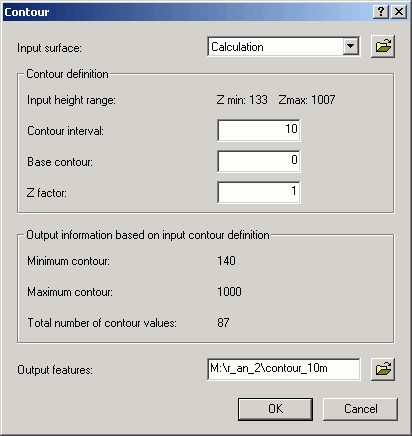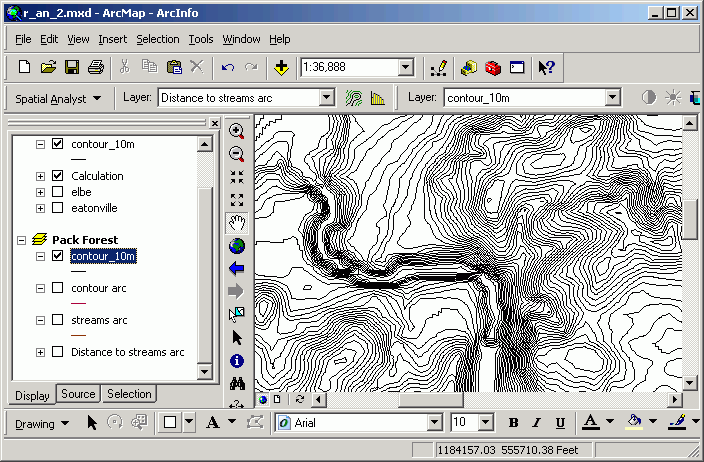Calculating summary attributes for features using a grid layer ("Zonal statistics ")

Zones in one grid layer can be defined by either polygons or zones of integer grids. For areas within different polygons, or for zones within an integer grid, the input grid values are summarized. The output is a table in which a single record exists for the unique values in the chosen field in the zone-defining layer. Each record in the output table contains the fields Area, Min, Max, Range, Mean, Std, and Sum.

In this example, the zone-defining layer is Stands. The individual zones are polygons containing the same value for the SITE_INDEX field. This means that for every unique occurrence of a site index value in the Stands layer, a new grid zone will be defined (even if the stands are not contiguous). The layer to be summarized is Dem.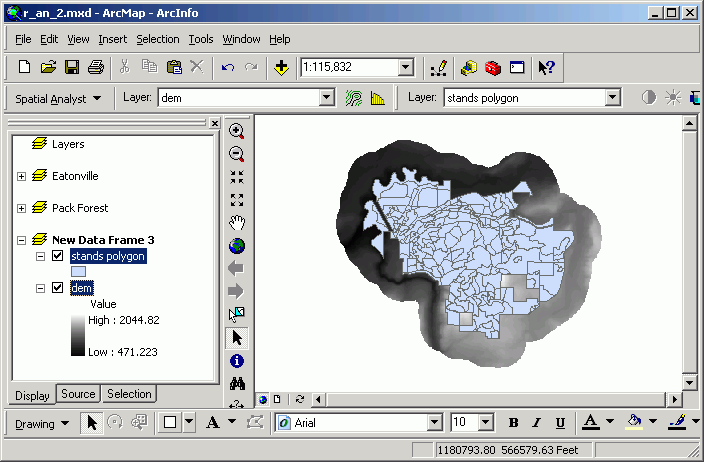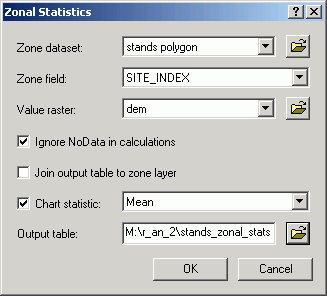The statistic shown on the graph is the Mean, that is, the mean elevation within each unique site index zone. In this case, each data marker in the graph signifies the mean elevation for all cells within stands with that site index.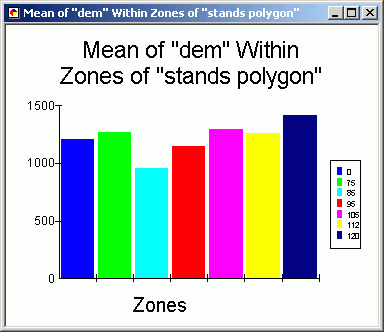Based on the graph, the stands with the greatest site index (a proxy measure for productivity) also have the highest mean elevation.

Cross tabulating areas

Cross-tabulation allows you to compare the area of one specific value in integer grid layer against one specific value in an another integer grid layer. The input layers and fields are defined in an ArcToolbox tool.

In this example, the Species field in the Stands layer is compared against the Soil.name field in the Soils layer.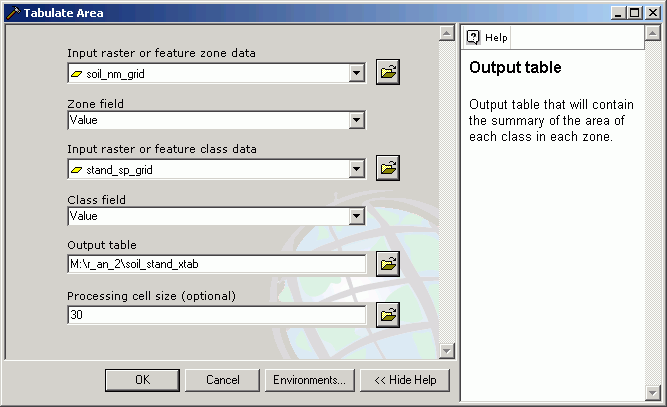The output table contains a unique value for each Species record, and fields representing unique values from the Soils layer.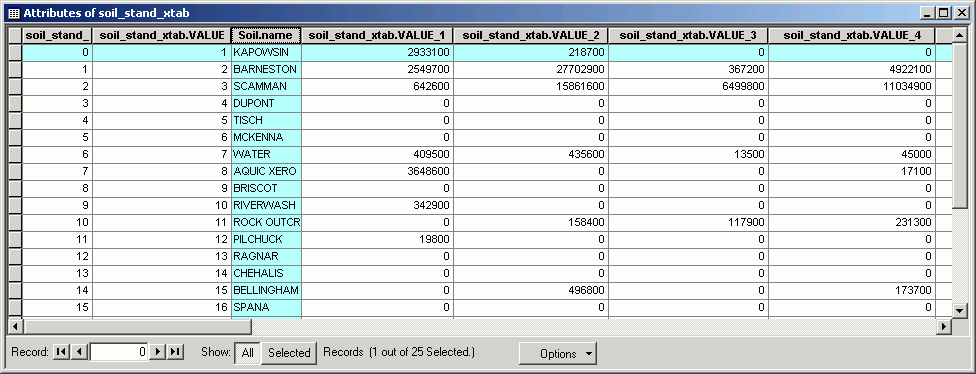Here is the stands grid table showing the values corresponding to the field names in the cross-tabulation table (i.e., Value = 1 corresponds to soil_stand_xtab.VALUE_1):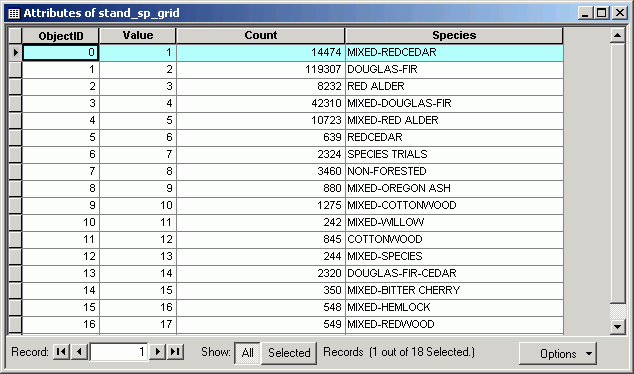The values in the fields are the area (in map units) for the spatial overlap between the classes in the input layers. For example, in Kapowsin soils, there are 2933100 ft^2 of Mixed Redcedar stands.

If you have two layers representing the same data for a study area at different times, you can use cross-tabulation for change analysis. Tabulation can be used any combination of (integer) grid layers.

Cross-tabulating areas is a raster analysis technique. When tabulating areas for polygon layers, you need to first convert from polygon to grid. You should select a cell size that will capture the detail of the features in the polygon data. The smaller the cell size, the greater the precision, but the longer processing will take..

This is a very powerful technique for change analysis. If you have datasets representing two different time slices, you can compare the area of such attributes as land cover or zoning designations.

"Querying" across multiple grid layers

While the normal feature attribute table query allows a query only on a single layer, the Raster Calculator allows you to make a complex query based on multiple layers. These types of queries are simple to perform as long as the grid layers representing the properties in question are contained in a single data frame. To do the same query in the vector world requires polygon layers representing the layers (which is in itself a problem, since vector layers are not good at representing continuous phenomena), and the performance of multiple topological overlay operations.

In this example, I am interested in finding cells closer than 300 ft from a stream, with an elevation > 1500, with greater than 6,000 bd-ft timber volume.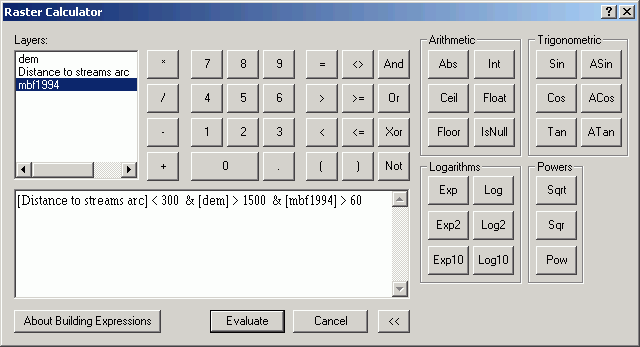Those cells displayed in green meet the criteria (coded with a value of 1).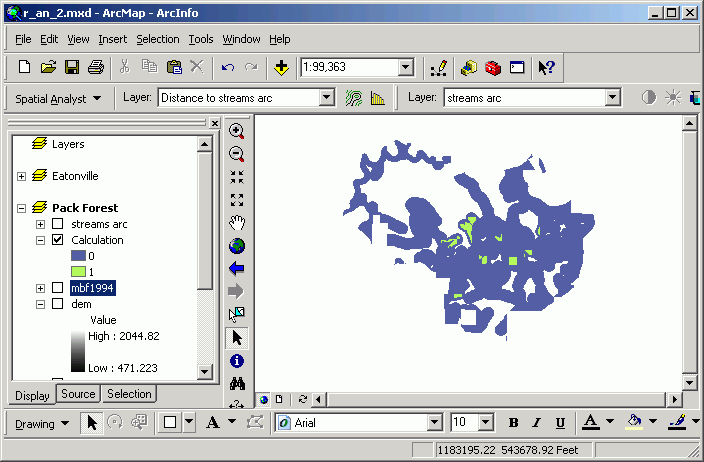Calculating neighborhood statistics

Neighborhood statistics are the focal functions referred to in Raster Analysis I. The neighborhood is defined as the group of cells for which statistics will be calculated. The neighborhood (a.k.a. kernel or focus) can be shaped as a circle, rectangle, ring, or wedge. Statistics available are

 Minimum Maximum Mean Median Sum Range Standard Deviation Majority Minority Variety

The processor looks in the neighborhood, identifies cells or point features within that neighborhood, and calculates a single statistic for that neighborhood. That single value is then placed in the output grid in the cell located at the center of the neighborhood. The process is performed for every input cell location in the analysis window.

It is possible to perform neighborhood statistics on point layers. If a point layer has a numeric field, the process is performed for the entire area within the analysis window, and the statistic is generated for the points located within the kernel at each output cell location.

A typical use of neighborhood statistics is known as "filtering." A "low pass" filter is nothing more than a 3 by 3 cell focal mean performed for an entire grid. Low pass filters smooth out anomalies and peaks in surfaces.

A "high pass" filter is also a 3 by 3 focal function, but rather than taking the mean of the 9-cell window, it performs a focal sum of the kernel cells, but first multiplies the cells by these coefficients: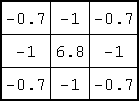There are several different coefficients that can be used in a high-pass filter, but they all have the objective of sharpening edges. ArcGIS 's default high-pass filter uses these particular coefficients.

In this example, an input grid represents several different vegetation zones (stand age).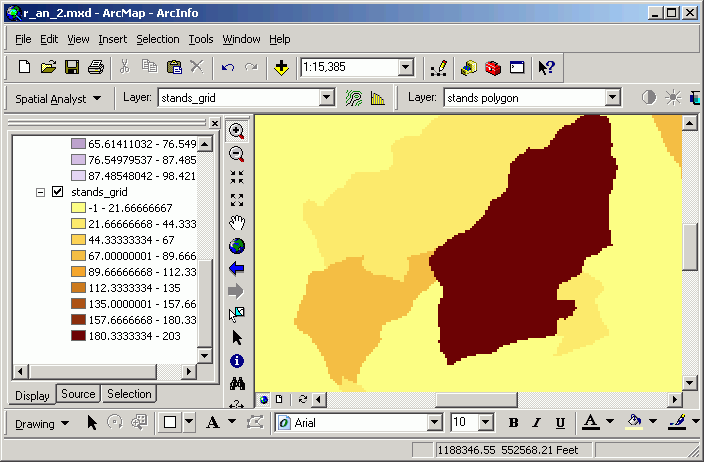The high pass filter makes the zone interiors the same value (0), while the edges get either a high or low value. The edges are most pronounced where the contrast is greatest.

This analysis is performed using the Filter tool: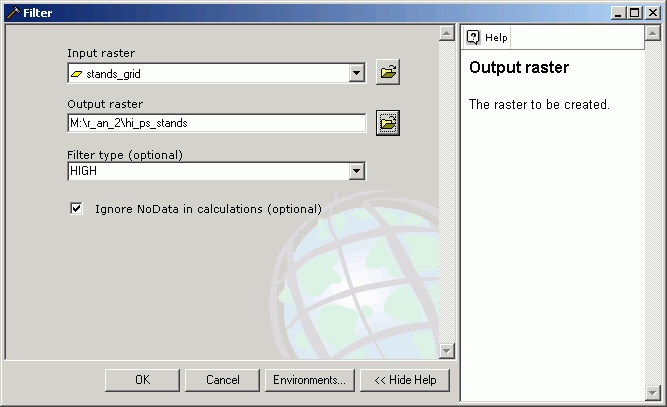With this grid as the result: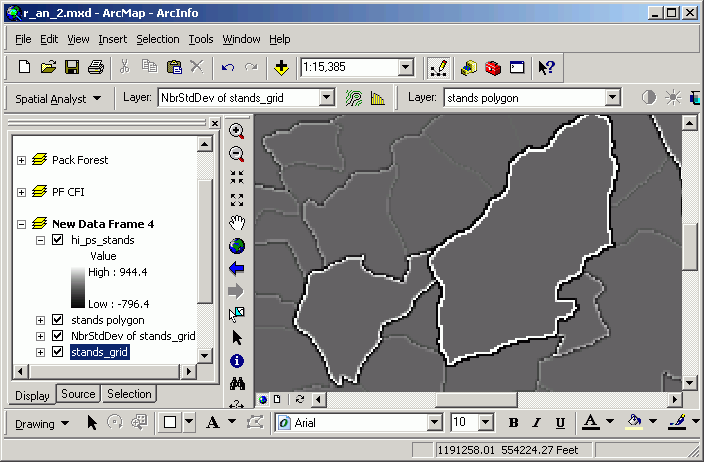Edges can be used to define places where animal movement may be hindered, or where species that prefer ecotones may be found.

Conditional processing

Conditional processing is a method of creating new grids based on an "if-then" condition. For example, we may be interested in reclassifying cells that have a certain value, but leaving other cells with their original value, this is possible with a reclassification. However, reclassification can be tedious (setting up the output classes), whereas conditional processing can create the new grid based on specific rules rather than simple numerical transformations. The conditions can also include several grids, rather than reclassifying based only on the values within a single grid.

Going back to the mosaicked Eatonville/Elbe grids, all cells between 500 and 700 m in elevation are multiplied by 100, and anything else is coded with a value of 0.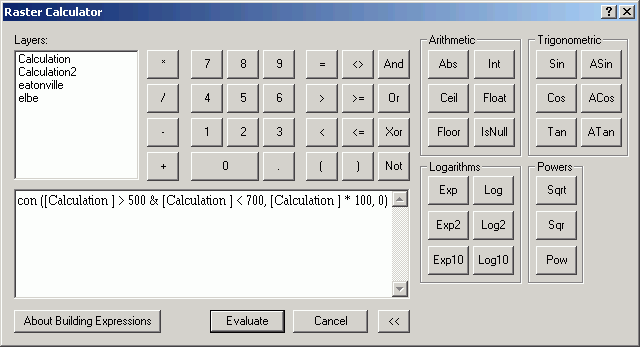con ([Calculation] > 500 & [Calculation] < 700, ~
[Calculation] * 100, ~
0)

The expression means this line-by-line, in English:

If elevation is greater than 500 and less than 1700, then
set output value to (elevation * 100), or else
set output value to 0

Here is the resultant grid: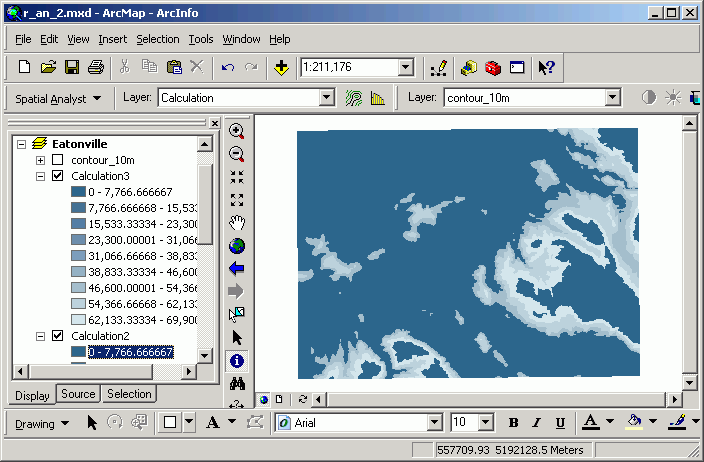Conditional processing is very useful when you need to select out or analyze a specific group of cells in one way, and another group of cells in another way.

Converting raster and vector data sources

It is possible to go back and forth between raster and vector formats. This always is at the expense of the loss, or generalization, of shapes. Any feature layer can be converted to a grid layer.

Vector to Raster:
Points are converted to single cells. Lines are converted to groups of cells oriented in a linear arrangement. Polygons are converted to zones. In all cases, only selected features are converted, or all features if no selection is active.

Raster to Vector:
Grid layers can only be converted directly to polygon vector layers. Be careful, because a new polygon will be created based on the field that is used for the conversion. If you have an elevation grid layer and you convert this to a polygon feature layer based on the Value field, you will get a very large number of very small polygons, and this will take a long time. It is more customary to first reclassify grids to create zones, and then convert these zones to polygon features.

Here, the Pack Forest dem has been reclassified into 100-ft elevation bands and then saved as a shape file. The value for the new polygon attribute Gridcode matches the original Value field from the input grid data source.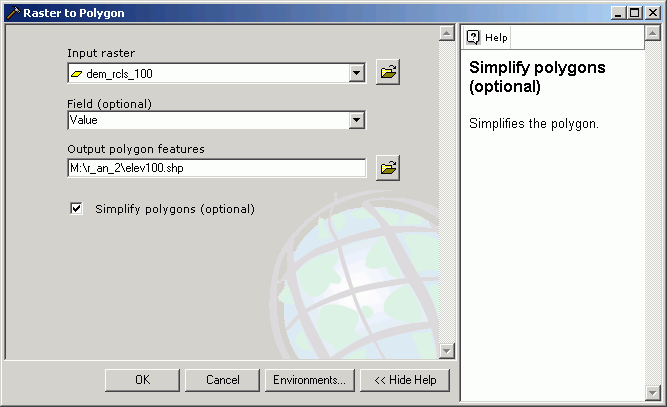The new polygon layer is displayed in a graduated color classification based on the Gridcode field.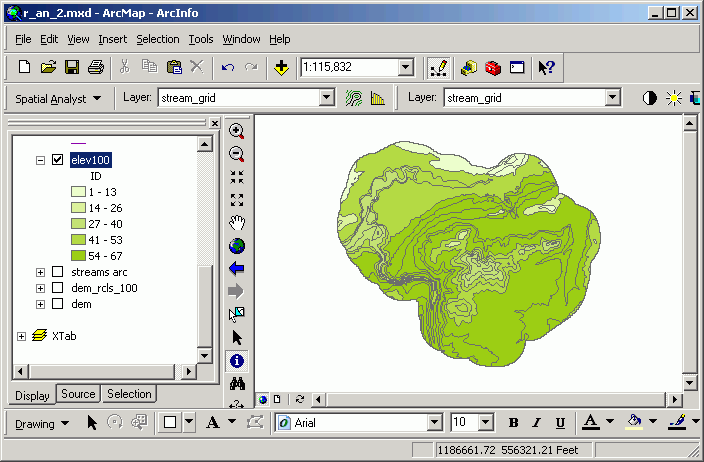Here, the streams line feature layer has been converted to a grid layer based on the DNR_TYPE field.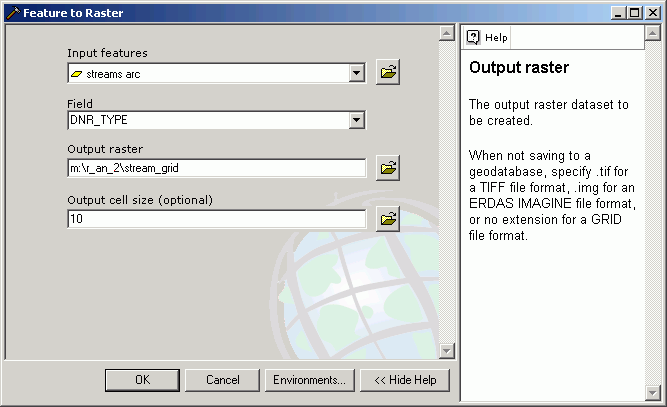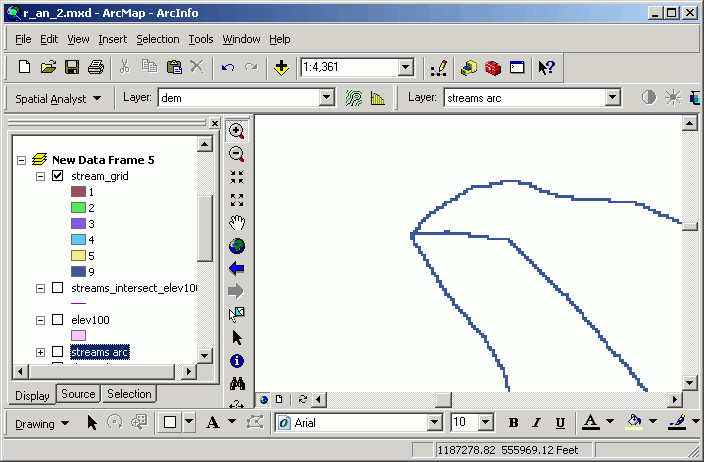Once a raster dataset has been converted to vector format, all of the vector analysis and overlay tools can be used. Likewise, when a vector datasets is converted to a grid, it can be used in raster analytical techniques.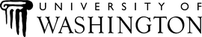The University of Washington Spatial Technology, GIS, and Remote Sensing Page is supported by the School of Forest Resources School of Forest Resources